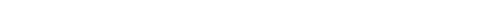## Question of the Week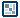Trigonometric Animations - Page 1
by kirupa  |  2 October 2009

This title may sound scary to you, and it probably isn't because of the word Animations. The word trigonometric to many of you brings up memories of school with random repeating graphics, cosine, sine, and Pi. Don't worry if you forgot everything about trigonometry or never had a chance to learn it. That is OK for today, for my goal with this article is to emphasize just the portions of trigonometry that make sense when used in animations.

For example, the following animation may just look like a bunch of circles just flying around after you mouse over the button:

Looking deeper, there is a bit more to it. This effect, dubbed the Colorful Explosion by me, uses some trigonometric magic. De-mystifying this magic is where this tutorial comes in. By the end of this article, you will have gained the ability to learn how the above and a range of other animations can be created using just trigonometric functions.

Basics of Trigonometric Motion (Possibly Boring)
There is really only one thing that characterizes trigonometric motion. That one thing is periodicity or, to put more simply, predictability. All trigonometric animations fall into a predictable pattern - almost too predictable actually.

For example, two common trigonometric functions you will be using are Cosine and Sine. These functions take a number as the input and return another number as the output. Regardless of how large or small the input is, the number that gets returned is always, by default, between -1 and 1: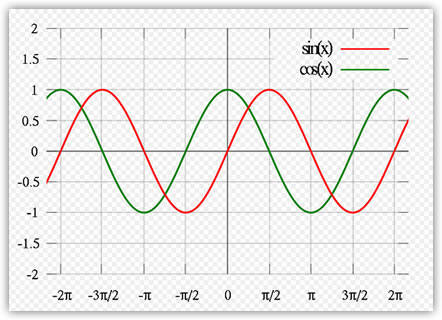[ image plotting Cosine and Sine taken from wikipedia ]

Let's look at the above chart in greater detail. The input to the function is an angle similar to what you see on the x-axis. The angle is in terms of radians, so that is why you see the Pi symbols. When the Cosine and Sine functions that the angle as the input, they return a value known as the amplitude. This amplitude is what is plotted on the y axis.

One characteristic of trigonometric animations I mentioned is periodicity. In the above graph, let's focus in on the Sine function. Notice that the range of angles shown in the graph goes from -2 Pi (-6.28) and 2 Pi (6.28). Despite the range of the angles, notice that the amplitude never deviates beyond -1 or 1. Even an angle of 1000 Pi would result in a value between -1 and 1.

The periodicity also means that you do not need to look over every possible angle to figure out what the value of your Cosine or Sine function will be. What you need to find is a single tile of your Cosine or Sine function that you can repeat forever without ever having lost any fidelity in your pattern.

For example, this is would be considered an example of such a tile: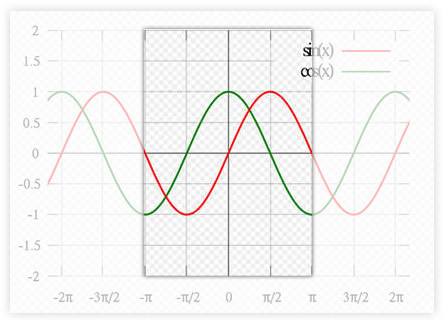This would be another example of a tile: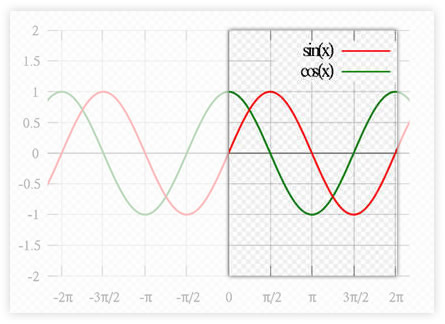For a simple cosine function, the tile's range of angles will be 2 PI. Something from negative PI to positive PI or something from 0 to 2 PI would all work because the range of angles between those endpoints contains 2 PI worth of them!

Ok, this page was a little dry. I will make up for this on the the next page where we jump right into some code.

Onwards to the next page.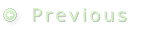1 | 2 | 3 | 4